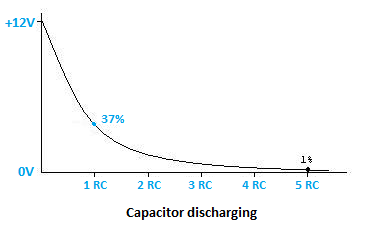# RC Time Constant Calculator

RC Time Constant Calculator will help you to easily calculate the time delay to reach required output voltage.

For calculations of NTC Thermistor Resistance please visit, NTC Thermsitor Resistance Calculator.

For calculations of Heat Sink Thermal Resistance please visit, Heat Sink Thermal Resistance Calculator.

Below is the RC Time Constant Calculator;

Enter the values of Vin, Vo, R and C in empty cells and then press Calculate to get the value of time delay.

### What is RC time constant?

When ever we charge a capacitor with a applied voltage level i.e. when input voltage is applied to the capacitor, it's take some time by the capacitor to set to that new applied voltage level.

Along with the capacitor, how much time it takes to set to new voltage level is defined also by the resistance of the circuit. Both resistance and capacitance plays important role in time delay to get output voltage in the circuit.

The RC time constant is a measure that helps us to know how long it will take a capacitor to charge to a certain or required voltage level.

To a capacitor of capacitance C if a voltage is applied through a resistor of resistance R, we can observe that the voltage across the capacitor rises slowly. Therefore, The time constant is defined as, the time requires by the capacitor to charge to 63.21% of the final output voltage value.

t = RC (seconds)

### RC time constant formula

The formula used to calculate the time delay is :

t = -log[(Vin-Vo)/Vin]R*C

To calculate output voltage Vo at a particular time, above formula can be re-write as :

Vo = Vin-{Vin*exp[-t/(R*C)]}

Where,

Vin = Applied input voltage
Vo = Output voltage to achieve
R = Resistance
C= Capacitance
t = Time delay

### RC time constant circuit

Below is the RC circuit which is getting charged from a 12V input voltage source;RC Time Constant Circuit

If we plot a graph we can observe that the capacitor charging started from 0V, and at 1st constant RC the capacitor will be charge up to 63% and at 5th constant RC it will get full charge.RC time constant for capacitor charging

After full charge of capacitor to 12V, if we taken out the input voltage supply i.e. OFF the supply, the capacitor starts to discharge. Now here also, the discharge time will be observed by the RC time constant.

Below graph shows the RC time constant of discharging of capacitor. We can observe at 1st constant RC the capacitor will be discharge up to 37% and at 5th constant RC it will get fully discharge.RC time constant for capacitor discharging

### Conclusion

RC time constant calculator will help you to find the delay time to get output voltage. This delay you can control by changing the value of resistance and capacitance. It will help you in controlling the signal delay or phase delay or other digital delays in a circuit for getting output or to get fast response.